Published © CC BY-SA

Gnome Forecaster

Our Gnome becomes a weather forecaster with an Arduino Nano Every and a pressure sensor. Get your local forecast without the internet.

BeginnerFull instructions provided1,345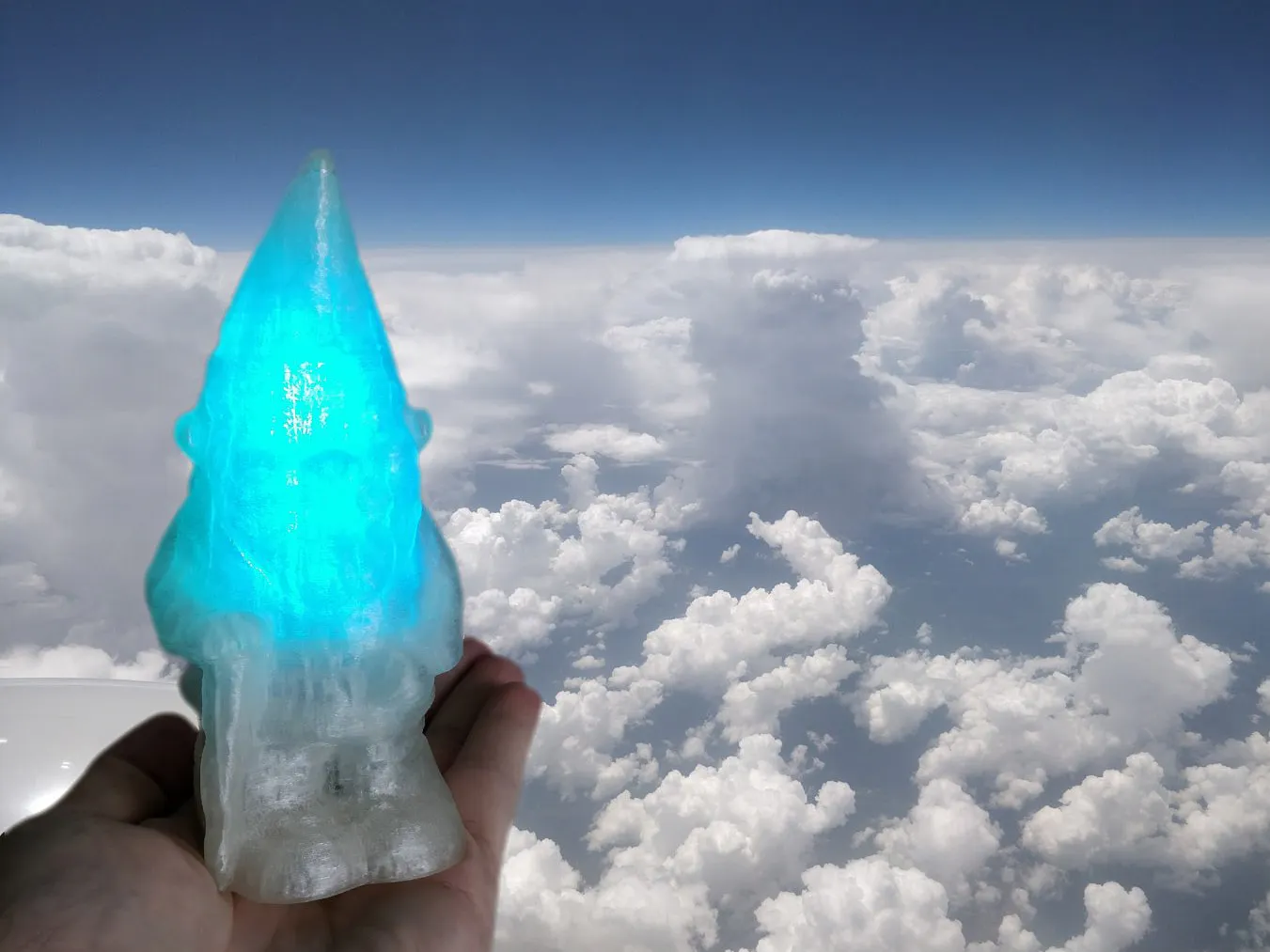Things used in this project

Hardware components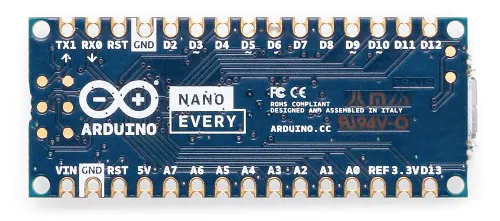Arduino Nano Every
×1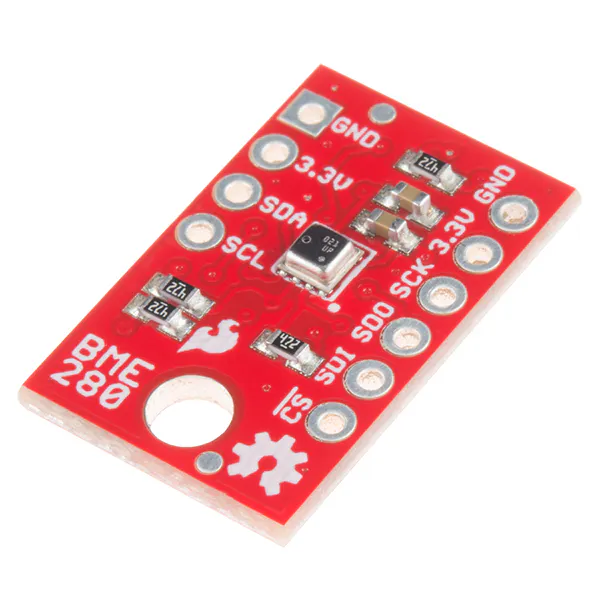SparkFun Atmospheric Sensor Breakout - BME280
×1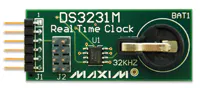Maxim Integrated DS3231MPMB1 Peripheral Module
×1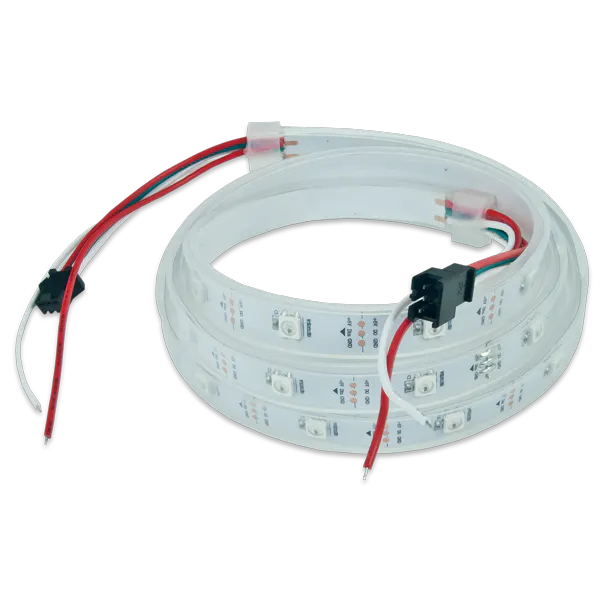Digilent WS2812 Addressable LED Strip
×1

Hand tools and fabrication machines3D Printer (generic)

Schematics

Complete circuit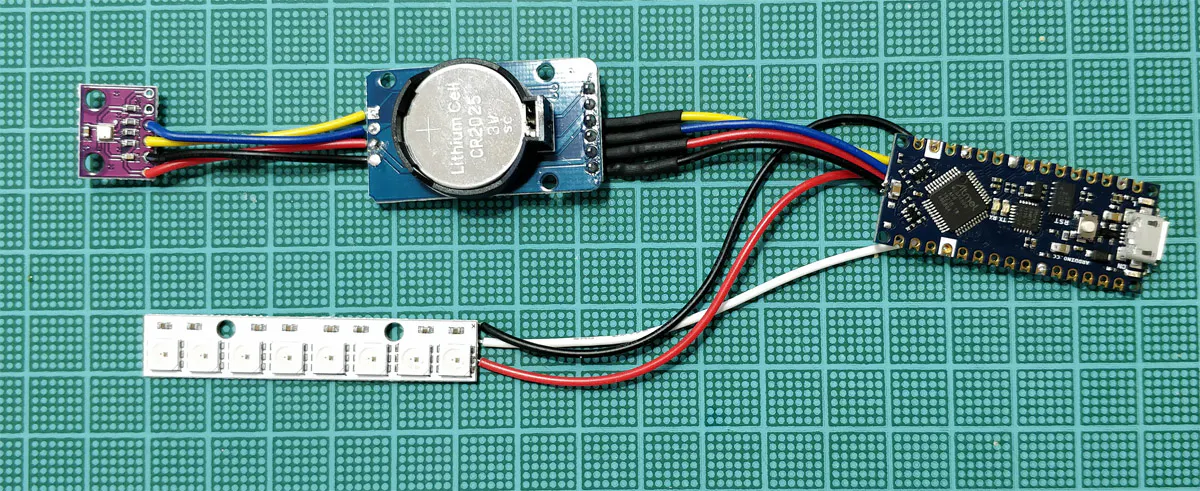Code

Gnome Weather Forecaster

Arduino
Open this sketch in your Arduino IDE and make sure you have downloaded all the libraries needed.
#include <Wire.h>
#include "RTClib.h"

#define SEALEVELPRESSURE_HPA (1013.25)
#define LED_PIN    7
#define NumLEDs 8

RTC_DS3231 rtc;   // initialise the RTC DS3231
Adafruit_BME280 bme;  // initialise the BME280 sensor
Adafruit_NeoPixel strip(NumLEDs, LED_PIN, NEO_GRB + NEO_KHZ800);

unsigned long delayTime;  // refresh rate for the readings
int t_hour = 0;
int t_minute = 0;
int oldpos = 0;     //Variables to scroll the LED bar
int newpos = 0;

int pressureArray = {0};  // here we store the pressure readings
byte counter = 0;
byte delta_time = 0;
int Z = 0;

char tStr;
char pStr;
char hStr;
char pseaStr;
char timeStr;
char dateStr;
char zambretti = "N/A";
char pressureHistory;
char pressureHistory2;

int temperature;
int humidity;
int pressure;
int altitude = 355;   // Here you should put the REAL altitude of the iGnome

void setup() {
bool status;
Serial.begin(57600);   // default settings
Serial.println("Starting measurements");
if (! rtc.begin()) {
Serial.println("Couldn't find RTC");
while (1);
}

if (rtc.lostPower()) {
Serial.println("RTC lost power, lets set the time!");
// following line sets the RTC to the date & time this sketch was compiled

}
status = bme.begin();
if (!status) {
while (1);
}
delayTime = 20000;

strip.begin();           // INITIALISE the LED strip object
strip.show();            // Turn OFF all pixels after init
strip.setBrightness(100); // Set BRIGHTNESS to about 2/5 (max = 255)
}

void loop() {

pressure = (int)(bme.readPressure() / 100.0F);

// Let's see the reading that will determine the forecast

Serial.print("Measured altitude ");

int seapressure = station2sealevel(pressure, altitude, temperature);

DateTime now = rtc.now();
int t_hour2 = now.hour();
int t_minute2 = now.minute();

if (t_hour2 != t_hour or t_minute2 != t_minute) {
delta_time++;
if (delta_time > 10) {    // every minute we increment delta_time, then every 10 minutes
delta_time = 0;         // we store the value in the array

if (counter == 10)  // if we read 10 values and filled up the array, we shift the array content
{
for (int i = 0; i < 9; i++) {   // we shift the array one position to the left
pressureArray[i] = pressureArray[i + 1];
}
pressureArray[counter - 1] = seapressure;
}
else {        // this code fills up the pressure array value by value until is filled up
pressureArray[counter] = seapressure;
counter++;
}
}

Z = calc_zambretti((pressureArray + pressureArray + pressureArray) / 3, (pressureArray + pressureArray + pressureArray) / 3, now.month());

// From here is just for debug purposes on the serial monitor

sprintf(zambretti, "Z=%d", Z);
sprintf(tStr, "%d C", temperature);
sprintf(hStr, "%d %%", humidity);
sprintf(pStr, "%d hPa", pressure);
sprintf(pseaStr, "%d hPa", seapressure);
sprintf(dateStr, "%02d.%02d.%d", now.day(), now.month(), now.year());
sprintf(timeStr, "%02d:%02d", now.hour(), now.minute());
sprintf(pressureHistory, "%d,%d,%d,%d,%d,%d,%d,%d,%d,%d,", pressureArray, pressureArray, pressureArray,
pressureArray, pressureArray, pressureArray, pressureArray, pressureArray, pressureArray, pressureArray);

Serial.println(zambretti);
Serial.println(tStr);
Serial.println(hStr);
Serial.println(pStr);
Serial.println(pseaStr);
Serial.println(dateStr);
Serial.println(timeStr);
Serial.println(pressureHistory);
Serial.println(pressureHistory2);

// END of testing purposes

StripScroll();    // advance the LED colors in the strip preparing for the new entry

if (pressureArray > 0 and pressureArray > 0) {
if (pressureArray + pressureArray + pressureArray - pressureArray - pressureArray - pressureArray >= 3) {
//RAISING
Serial.println("Raising");
if (Z < 3) {
Serial.println("Sunny");
strip.setPixelColor(0, 255, 127, 0);
}
else if (Z >= 3 and Z <= 9) {
Serial.println("Sunny Cloudy");
strip.setPixelColor(0, 0, 255, 255);
}
else if (Z > 9 and Z <= 17) {
Serial.println("Cloudy");
strip.setPixelColor(0, 127, 0, 255);
}
else if (Z > 17) {
Serial.println("Rainy");
strip.setPixelColor(0, 255, 0, 127);
}
}

else if (pressureArray + pressureArray + pressureArray - pressureArray - pressureArray - pressureArray >= 3) {
//FALLING
Serial.println("Falling");
if (Z < 4) {
Serial.println("Sunny");
strip.setPixelColor(0, 255, 127, 0);
}
else if (Z >= 4 and Z < 14) {
Serial.println("Sunny Cloudy");
strip.setPixelColor(0, 0, 255, 255);
}
else if (Z >= 14 and Z < 19) {
Serial.println("Worsening");
strip.setPixelColor(0, 192, 0, 255);
}
else if (Z >= 19 and Z < 21) {
Serial.println("Cloudy");
strip.setPixelColor(0, 127, 0, 255);
}
else if (Z >= 21) {
Serial.println("Rainy");
strip.setPixelColor(0, 255, 0, 127);
}
}
else {
if (Z < 5) {
Serial.println("Sunny");
strip.setPixelColor(0, 255, 127, 0);
}
else if (Z >= 5 and Z <= 11) {
Serial.println("Sunny Cloudy");
strip.setPixelColor(0, 0, 255, 255);
}
else if (Z > 11 and Z < 14) {
Serial.println("Cloudy");
strip.setPixelColor(0, 127, 0, 255);
}
else if (Z >= 14 and Z < 19) {
Serial.println("Worsening");
strip.setPixelColor(0, 192, 0, 255);
}
else if (Z >= 19) {
Serial.println("Rainy");
strip.setPixelColor(0, 255, 0, 127);
}
}
}
else {
if (seapressure < 1005) {
Serial.println("Rainy");
strip.setPixelColor(0, 255, 0, 127);
}
else if (seapressure >= 1005 and seapressure <= 1015) {
Serial.println("Cloudy");
strip.setPixelColor(0, 127, 0, 255);
}
else if (seapressure > 1015 and seapressure < 1025) {
Serial.println("Sunny Cloudy");
strip.setPixelColor(0, 0, 255, 255);
}
else {
Serial.println("Rainy");
strip.setPixelColor(0, 255, 0, 127);
}
}

t_hour = t_hour2;
t_minute = t_minute2;

}
strip.show();
delay(delayTime);

}

int calc_zambretti(int curr_pressure, int prev_pressure, int mon) {
if (curr_pressure < prev_pressure) {
//FALLING
if (mon >= 4 and mon <= 9)
//summer
{
if (curr_pressure >= 1030)
return 2;
else if (curr_pressure >= 1020 and curr_pressure < 1030)
return 8;
else if (curr_pressure >= 1010 and curr_pressure < 1020)
return 18;
else if (curr_pressure >= 1000 and curr_pressure < 1010)
return 21;
else if (curr_pressure >= 990 and curr_pressure < 1000)
return 24;
else if (curr_pressure >= 980 and curr_pressure < 990)
return 24;
else if (curr_pressure >= 970 and curr_pressure < 980)
return 26;
else if (curr_pressure < 970)
return 26;
}
else {
//winter
if (curr_pressure >= 1030)
return 2;
else if (curr_pressure >= 1020 and curr_pressure < 1030)
return 8;
else if (curr_pressure >= 1010 and curr_pressure < 1020)
return 15;
else if (curr_pressure >= 1000 and curr_pressure < 1010)
return 21;
else if (curr_pressure >= 990 and curr_pressure < 1000)
return 22;
else if (curr_pressure >= 980 and curr_pressure < 990)
return 24;
else if (curr_pressure >= 970 and curr_pressure < 980)
return 26;
else if (curr_pressure < 970)
return 26;
}
}
else if (curr_pressure > prev_pressure) {
//RAISING
if (mon >= 4 and mon <= 9) {
//summer
if (curr_pressure >= 1030)
return 1;
else if (curr_pressure >= 1020 and curr_pressure < 1030)
return 2;
else if (curr_pressure >= 1010 and curr_pressure < 1020)
return 3;
else if (curr_pressure >= 1000 and curr_pressure < 1010)
return 7;
else if (curr_pressure >= 990 and curr_pressure < 1000)
return 9;
else if (curr_pressure >= 980 and curr_pressure < 990)
return 12;
else if (curr_pressure >= 970 and curr_pressure < 980)
return 17;
else if (curr_pressure < 970)
return 17;
}
else
//winter
{
if (curr_pressure >= 1030)
return 1;
else if (curr_pressure >= 1020 and curr_pressure < 1030)
return 2;
else if (curr_pressure >= 1010 and curr_pressure < 1020)
return 6;
else if (curr_pressure >= 1000 and curr_pressure < 1010)
return 7;
else if (curr_pressure >= 990 and curr_pressure < 1000)
return 10;
else if (curr_pressure >= 980 and curr_pressure < 990)
return 13;
else if (curr_pressure >= 970 and curr_pressure < 980)
return 17;
else if (curr_pressure < 970)
return 17;
}
}
else {
if (curr_pressure >= 1030)
return 1;
else if (curr_pressure >= 1020 and curr_pressure < 1030)
return 2;
else if (curr_pressure >= 1010 and curr_pressure < 1020)
return 11;
else if (curr_pressure >= 1000 and curr_pressure < 1010)
return 14;
else if (curr_pressure >= 990 and curr_pressure < 1000)
return 19;
else if (curr_pressure >= 980 and curr_pressure < 990)
return 23;
else if (curr_pressure >= 970 and curr_pressure < 980)
return 24;
else if (curr_pressure < 970)
return 26;

}
}

int station2sealevel(int p, int height, int t) {  // from pressure at our height to sea level
return (double) p * pow(1 - 0.0065 * (double)height / (t + 0.0065 * (double)height + 273.15), -5.275);
}

void StripScroll() {    // scroll up all the LED strip by 1
for (int led = 0; led <= (NumLEDs); led++)
{
oldpos =  NumLEDs - led - 2;
newpos = oldpos + 1;
strip.setPixelColor(newpos, strip.getPixelColor(oldpos));
}
}

Credits

Arduino_Genuino

70 projects • 6213 followers